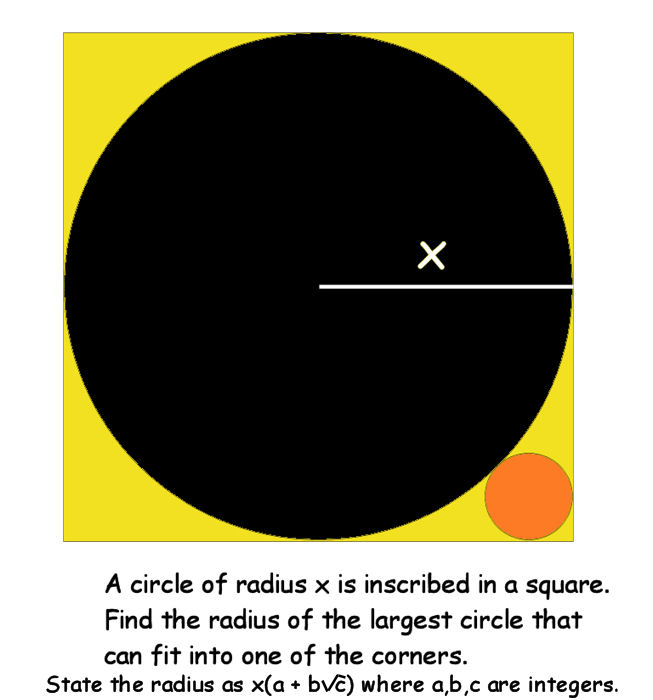# How big is the orange?

Geometry Level 3A circle of radius x is inscribed in a square. What is the radius $r$ of the largest circle that can fit into one of the corners?

State the radius as $r = x(a + b \sqrt{c})$ where $a, b, c,$ are integers and $c$ is not divisible by the square of any prime. Find $a+b+c$.

×